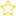See the live dashboard for CensusAtSchool 2021

These lesson plans were developed in conjunction with the TLRI research project: Building students’ inferential reasoning: statistics curriculum levels 5 and 6.

While each series of activities are set up as lesson plans, they may take more or less than a lesson of class time.  They form a possible sequence of learning and each block works together with the other blocks.  The lessons are developed using CensusAtSchool (2009) data, they could be adapted to any data set or any year of CensusAtSchool.

Lesson 5: Introducing sampling

Focus for lesson: • Revisit posing investigative questions, some summary mostly comparison. • Identifying and clarifying the population • Sampling variability – all samples from a given population are different (can’t tell exactly) • Distorted view of population – samples give (same) distorted view, similarity among samples • What is a sample? • Why sample?

Lesson 6: Introducing informal inferential reasoning

Focus for lesson: • Can the sample tell us something about the population? • Informal inferential reasoning • Making I wonder statements about the population.

Lesson 7: Introduction to box plots

Focus for lesson: • Introducing the idea of middle group and overall spread. • Describing the position of middle groups relative to one another. • Describing spread of middle group and spread overall.

Lesson 8: Formalising, description and inference – box plots

Focus for lesson: • Drawing box plots by finding medians and quartiles from dot plots. • Describing box plots making I notice statements. • Inferring from box plots making I wonder statements. • Focus on the language of description and inference.

Resources:

• Event: 2009 Statistics Teachers' Day
• Keywords: Box plots, Five Number Summary, Inference, Sampling
• (Last updated: 15/05/19. Added: 20/11/12)
• Rate this resource:(No ratings yet)